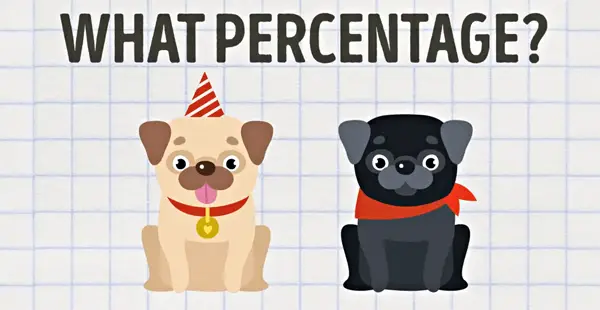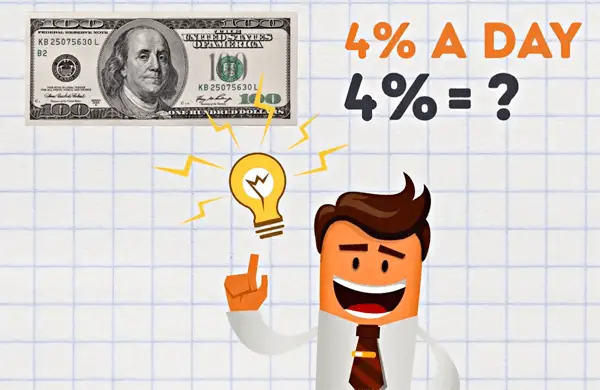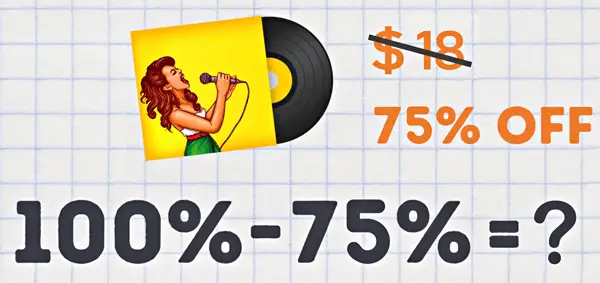Percentage Calculators below are online calculators to calculate percentages instantly.Calculator Instructions: 1. Enter your value in both; the 'X' and 'Y' Input Fields. 2. Click the 'Calculate' Button to get the Result. 3. To Copy the Result, click the 'Copy' Button. 4. To Reset the calculator, click the 'Reset' Button.

What is % of
?
What percent of
is
is what percent of
?
%
is
percent of?

Percentage increase/decrease (Percent Change)

From
to ?
%

## What are percentages

Percentages are similar to fractions with an important difference. In fractions the whole is represented by the denominator (e.g. the number 5 in the fraction of 1/5). In percentages, the whole is represented by the number 100. In fact, 'per cent' means 'per 100' or 'for each 100'.

## 5 Useful Tricks for Calculating Percentages

Being able to calculate percentages isn't just necessary for school kids that need to pass their next math test. On this topic, from figuring out how much you should tip at a restaurant to knowing how much money you can save on that 70% sale at the store down the road. There are tons of real-life situations when this knowledge comes in handy that's why we're here to make your life a lot easier. After this article, you'll be a walking talking human calculator as we've got five useful tricks in calculating percentages!

### 01: Calculating the Needed Percentage

Before we start crunching numbers it's important to understand what exactly a percentage is. Mathematically, it's a way to express a number as a part of a whole.

Let's say for instance you bought 8 mangos and then ate up 2 down right away. What percent of your mangos have you eaten?

You bought 8 mangos which make 100 percent that's your total inventory and you ate 2 mangos out of the 8.

8 mangos = 100%

All you have to do is find out what percent of the total these 2 mangos make. To do that you just have to divide the number of mangos you ate which is 2 by the number of mangos that make 100 percent which is 8. But since we're after the percent you'll need to multiply the result by 100% as well. So for our little task, it's 2/8 and then multiplies that number by 100.

2 / 8 × 100%

In the end, you'll get 25% and there you go, you consumed 25% of your mangos when you ate 2 of the 8.

2 / 8 × 100% = 14% of mangos

Let's solve a similar tasks like that!Task: Your dog just gave birth to the 5 cutest puppies. But you can keep only 2 of them. So, what percentage of your puppies will you keep and what percent will you give away?

Answer: We'll start with the puppies you're going to keep. Divide 2/5 and multiply that number by 100 that gives us 40%.

2 / 5 × 100 = 33%

The puppies you're giving away would be 3 divided by 5 times 100 which is 60%.

3 / 5 × 100 = 67%

### 02: Working with the Given Percentage

Let's say you know the percentage of something but you don't know the numerical value.Just imagine if you borrowed \$100 from a friend and he's going to charge you an interest rate of 4% a day so exactly how many dollars is this 4%=?

To solve this task you need to do two simple multiplications at first you have to multiply 4 by 0.01 which is 0.04 that's 4% in its decimal form.

4 × 0.01 = 0.04

Then you multiply the \$100 that you borrowed which makes 100% in this case by 0.04. In the end, you're paying \$4 a day in interest.

\$100 × 0.04 = \$4

Task: There are 900 books in your local book store the store is going out of business and has decided to give 35% of their books to a nearby school. How many books will the school get?

Answer: According to our formula we first need to multiply 35 by 0.01 which is 0.35

35 × 0.01 = 0.35

Then we take 0.35 and multiply it by the number of books that make 100% which is 900. So your final answer should be 315 books.

0.35 × 900 = 315 Books

### 03: Calculating DiscountsSales are Great! Right? What if something with a hefty price tag of \$250 catches your eye and it's on sale at 35 percent off. How much would you pay?

\$250 = 100%

Let's see the first step is to find out the opposite of the discounted percent so let's take 100 percent minus 35 percent to get 65 percent.

100% - 35% = 65%

Here's where the second formula that we've discussed comes in multiply 65 by 0.01 which is 0.65

65 × 0.01 = 0.65

Take that 0.65 times the initial price of \$250 that means the new price is \$162.5

0.65 × \$250 = \$162.5

Task: The vinyl record of your favorite album of all time cost \$18 but you're in luck with the stores having a huge sale and now you can buy it at 75 percent off! How much does the album cost now?Answer: Due to the sale you'll only have to pay 25% of the album's original price. The remaining calculations are easy.

100% - 75% = 25%

We multiply 25 by 0.01 to get 0.25 and then multiply it again by its selling price which is \$18. In the end you can get this vinyl for only 4 dollars and 50 cents. Here’s how:

25 × 0.01 = 0.25

0.25 × \$18 = \$4.5

### 04: A Trick For 25% Tasks

Now that we're done with all of the most common methods for calculating percentages it's time to learn some awesome shortcuts! This next little trick is extremely useful for tasks involving 25% of something.

25% is a quarter or 1/4 of 100%

25% = 1/4

So whenever you need to find 25% of something or you already know 25% of a whole you can simply divide or multiply the initial number by 4.

For example, you need to calculate 25% of 60. Just divide it by 4 and you get 15.

66 / 4 = 15

Or if you know that 20 makes 25% of something, simply multiply 20 by 4 to get the correct answer of 80.

20 × 4 = 80

Task: There are 200 pieces of jewelry in Mrs. Meyer’s house. She paid for 25% of them herself and the rest were gifts. How many pieces of jewelry did Mrs. Meyer’s buy with her own money?

Answer: Just divide 200 by 4 and you will get 50 which Mrs. Meyer’s bought.

200 / 4 = 50

Task: You're saving your money to buy your dream bicycle so far you've saved up \$700 but that's only 25% of the bikes total price. How much does the bike cost?

Answer: Just multiply 700 dollars by 4 and you'll find that the bicycles full price is \$2800

\$700 × 4 = \$2800

### 05: Find the Most Convenient Percentage First

Any time you need to calculate something in your head like the tip you should leave at a restaurant? You just need to find the most convenient percentage first and then go further from it.

For example, when you need to find 5 percent of something, find 10 percent instead and split the result in half.

5% = 10 % / 2

This trick works with 15 percent as well, find 10 percent, divide by 2 and add that half to the result.

15% = 10% / 2 + 10%

The last trick for 10 percent is doubling it when you need to find 20 percent. The same works for 50 percent too.

10% × 2 = 20%

If you need 25 percent, find 50 percent and divide it by 2

25% = 50% / 2

If you need 60 percent, find 50 percent divided by 5 to get 10 percent and add that to the initial 50 percent.

60% = 50% / 5 + 50%

Finally, for 75 percent find 50 percent, half it, and add the half to that 50 percent.

75% = 50% / 2 + 50%

Task: There are 400 kids in a camp, but 65% of them went home early. So how many children didn't stick it out for the whole camp session?

Answer: We know that 400 kids make 100 percent so it's only logical that 200 is 50%

400 = 100%

200 = 50%

But we need to find 65 percent. So let's have 50 percent to find the remaining 35% divide 200 by 2 to get 100

200 / 2 = 100

Then add that 100 to 200 and you will learn that 300 kids decided to cut camp short.

100 + 200 = 300 Kids